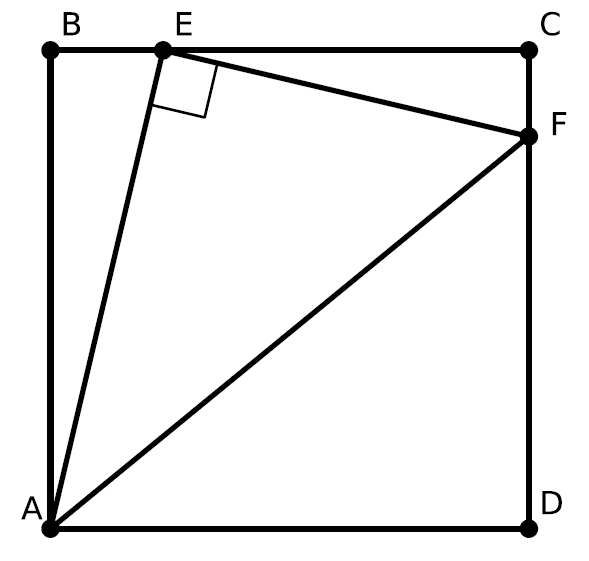# Right Triangle Tucked Into A Square

Geometry Level 1In the drawing above, $ABCD$ is a square. Points $E$ and $F$ are on $BC$ and $CD$ such that $AEF$ is a right triangle with $AE=4$ and $EF=3$. What is the area of the square?

×